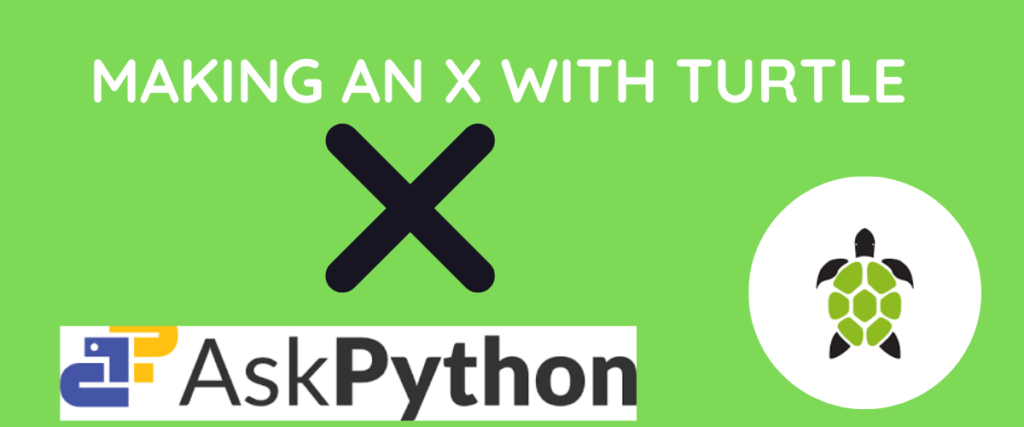# Drawing an ‘X’ Using Turtle- A Simple GuideTurtle is a famous Python module that lets you have some fun with graphics. It is most widely used to design graphic User interface (GUI) applications. To put it simply, with Turtle, we can draw shapes, pictures, and even logos and customize them with different colors and sizes.

Turtle is an animation and graphics development module that is part of Python’s standard library. It helps in drawing complex shapes and animations with a few simple functions.

Like Tkinter, turtle is also used to create graphical windows and animations.

Say Hello to Turtle!

## Turtle – Introduction

We have already understood that Turtle is used to draw graphics and various shapes and make them interactive. The instrument, or, as we can say, a pen, that is used to draw the illustrations is called the turtle. We can change the size, shape, and even color of the turtle.

We can also create a screen for displaying the illustrations in a grid. We don’t need to install it; it is pre-installed, and we can import it as a module.

Drawing shapes or illustrations using the turtle is typically based on us giving directions to the turtle(cursor) such as moving forward, backward, left, or right. We can also provide coordinates for a more simplified and systematic code.

Visit this article to learn how to set a starting position in Turtle

All we need to do is import the module, create an instance for the module, and boom! Our playground is all set to experiment.

Let us have some fun with our little turtle friend!

## Importing the Turtle Module

Since we don’t need to install the library, we can just import Turtle into our environment to use it. The command to import the module is given below.

```import turtle
```

## Drawing X With Turtle

Follow this simple code to draw the letter X on the screen.

First, let us import the module and create an instance for it.

```
```
```import turtle
t = turtle.Turtle()
```

As you can see, we have created an instance for the turtle. Now this cursor will move according to the directions we give in the following code.

```t.pensize(40)
```

Here, we are defining the thickness of the pen used to draw.

```t.left(60)
t.forward(100)
```

Here, we are making the cursor move 60 steps to the left and 100 in the forward direction. This makes a slant line (the half of X-`/`).

Now, from the mid-point of the line, we are going to move upwards and draw another line to create `Y`.

```t.back(50)
t.left(60)
```

Now all we have to do is draw another line away from the midpoint to create an X.

```t.forward(50)
t.backward(100)
turtle.done()
```

You can change the thickness of the illustration by modifying the pen size.

You can also change the cursor to an actual turtle shape.

```t = turtle.Turtle()
t.shape("turtle")
```

Here’s how you can change the color and thickness of the illustration.

```import turtle
t = turtle.Turtle()
t.shape("turtle")
t.color("green")
t.pensize(20)
t.left(60)
t.forward(100)
t.back(50)
t.left(60)
t.forward(50)
t.backward(100)
t.done()
turtle.done()
```

So that’s it! That is how you can draw an X with turtle and even customize it with just a few lines of code.

## Conclusion

To summarize, the Turtle is a Python module used to draw basic shapes, draw logos, and make beginner-friendly simple animations. Initially developed to introduce programming to kids with just two methods – turtle.forward() and turtle.right(), became the most popular tool for making simple GUI animations and drawings. We don’t need to install it separately. It comes installed with the Python installation itself so we can just import it into our environment.

In this article, we have seen the introduction to the turtle module, how to import it, and a small use case- drawing an X.

While drawing with turtle is mostly based on directions we give to the turtle cursor, we can also customize the colors, shapes,s and sizes of the drawings.

In this tutorial, we have seen two approaches one with thick lines and black color which is the basic implementation, and in the second one, we have learnt how to change the size of the pen, color of the drawing, and even the shape of the cursor.

## References

You can find more about Turtle here